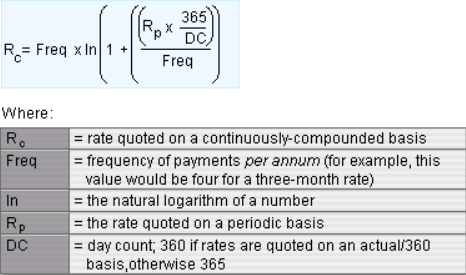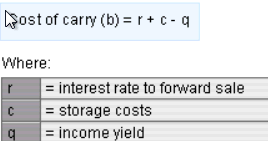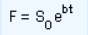Forward Pricing

We tend to use continuous rate to calculate forward price because it’s hard to compare forward price if market convention is different. For example, a 4% three-month rate in US dollar market is not the same as a 4% three-month rate in the UK sterling market. This is because USD ear fractions are calculated on an actual/360 basis while GBP rates are calculated on an actual/365 basis.

Here is the formula to convert market rates to continuous rates:For investment assets, interest rates and storage cost increase forward price, while income reduces forward price. The overall relationship is called the cost of carry for an asset.The overall future price equation, if all rates are quoted using a continuously-compounded basis, is: